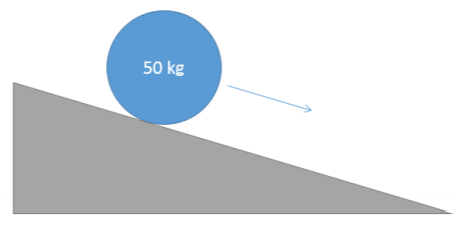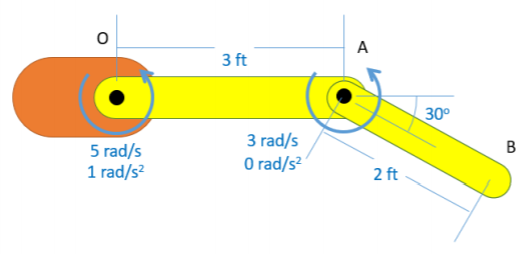# 12.5: Chapter 12 Homework Problems

$$\newcommand{\vecs}{\overset { \rightharpoonup} {\mathbf{#1}} }$$ $$\newcommand{\vecd}{\overset{-\!-\!\rightharpoonup}{\vphantom{a}\smash {#1}}}$$$$\newcommand{\id}{\mathrm{id}}$$ $$\newcommand{\Span}{\mathrm{span}}$$ $$\newcommand{\kernel}{\mathrm{null}\,}$$ $$\newcommand{\range}{\mathrm{range}\,}$$ $$\newcommand{\RealPart}{\mathrm{Re}}$$ $$\newcommand{\ImaginaryPart}{\mathrm{Im}}$$ $$\newcommand{\Argument}{\mathrm{Arg}}$$ $$\newcommand{\norm}{\| #1 \|}$$ $$\newcommand{\inner}{\langle #1, #2 \rangle}$$ $$\newcommand{\Span}{\mathrm{span}}$$ $$\newcommand{\id}{\mathrm{id}}$$ $$\newcommand{\Span}{\mathrm{span}}$$ $$\newcommand{\kernel}{\mathrm{null}\,}$$ $$\newcommand{\range}{\mathrm{range}\,}$$ $$\newcommand{\RealPart}{\mathrm{Re}}$$ $$\newcommand{\ImaginaryPart}{\mathrm{Im}}$$ $$\newcommand{\Argument}{\mathrm{Arg}}$$ $$\newcommand{\norm}{\| #1 \|}$$ $$\newcommand{\inner}{\langle #1, #2 \rangle}$$ $$\newcommand{\Span}{\mathrm{span}}$$$$\newcommand{\AA}{\unicode[.8,0]{x212B}}$$

Exercise $$\PageIndex{1}$$

The SUV shown below has an initial velocity of 90 ft/s. It slams on its brakes, coming to a stop over a distance of 300 feet. If the car has a weight of 3500 lbs and a center of mass as shown below, what are the normal forces at the front wheels? What are the normal forces at the back wheels?Figure $$\PageIndex{1}$$: problem diagram for Exercise $$\PageIndex{1}$$. A car traveling in a straight line applies its brakes, coming to a gradual stop.
Solution

$$F_{N_{rear}} = 1291.4 \ lbs$$

$$F_{N_{front}} = 2208.6 \ lbs$$

Exercise $$\PageIndex{2}$$

A ring-shaped space station can be approximated as a thin ring 60 meters in diameter with a mass of 500,000 kg. The space station has a set of thrusters able to exert equal and opposite forces as shown below. If we want to cause an angular acceleration of 0.1 rad/s² in the space station, what is the force required from each thruster?Figure $$\PageIndex{2}$$: problem diagram for Exercise $$\PageIndex{2}$$. A ring-shaped space station is given the specified angular acceleration through the firing of two thrusters located on either side of a diameter, pointing in opposite directions.
Solution

$$F_{thruster} = 750 \ kN$$

Exercise $$\PageIndex{3}$$

A 50-kg barrel with a diameter of 0.75 meters is placed on a 20-degree slope. Assuming the barrel rolls without slipping, what will the acceleration of the barrel's center of mass be?Figure $$\PageIndex{3}$$: problem diagram for Exercise $$\PageIndex{3}$$. A 50-kg barrel with a 0.75-meter diameter rolls down a 20° incline without slipping.
Solution

$$a_x = 2.24 \ m/s^2$$

Exercise $$\PageIndex{4}$$

A 3-meter-long, 25-kg beam is supported by two cables as shown below. You can treat the beam as a slender rod. Assume that we want the left end of the beam at point A to remain at a constant height while the right end of the beam at point B accelerates upwards at a rate of 1 m/s².

• What is the rate of acceleration of the center of the beam and the rate of angular acceleration for the beam?
• What will $$T_1$$ and $$T_2$$ need to be to achieve these accelerations?Figure $$\PageIndex{4}$$: problem diagram for Exercise $$\PageIndex{4}$$. A horizontal beam is held in the air by the tension forces from two vertical cables attached near the beam's ends.
Solution

$$a_{C\y} = 0.5 \ m/s^2, \, \alpha = 0.333 \ \frac{rad}{s}$$

$$T_1 = 81.75 \ N, \, T_2 = 176 \ N$$

Exercise $$\PageIndex{5}$$

You are modeling the robotic arm shown below. Treat each section of the arm as a slender rod. Section OA weighs 30 lbs and section AB weighs 18 lbs. If we want the relative angular accelerations and velocities shown below, what should the motor torques be at O and A? (This is a top-down view of the robot arm.)Figure $$\PageIndex{5}$$: problem diagram for Exercise $$\PageIndex{5}$$. Top-down view of a two-segment robotic arm with one end attached to a fixed base, with motors at the two joints providing rotation.
Solution

$$M_O = - 3.9 \ ft$$-$$lbs$$

$$M_A = -19.3 \ ft$$-$$lbs$$

This page titled 12.5: Chapter 12 Homework Problems is shared under a CC BY-SA 4.0 license and was authored, remixed, and/or curated by Jacob Moore & Contributors (Mechanics Map) via source content that was edited to the style and standards of the LibreTexts platform; a detailed edit history is available upon request.